# simdatasetreg

simdatasetReg simulates a regression dataset given the parameters of a mixture regression model

## Syntax

• y=simdatasetreg(n, Pi, Beta, S, Xdistrib)example
• y=simdatasetreg(n, Pi, Beta, S, Xdistrib,Name,Value)example
• [y,X]=simdatasetreg(___)example
• [y,X,id]=simdatasetreg(___)example

## Description

[y,X,id]=simdatasetreg(n, Pi, Beta, S) generates a regression dataset of size n from a mixture model with parameters 'Pi' (mixing proportions), 'Beta' (matrix of regression coefficients), and 'S' (vector of variances of the distributions of the points around each regression hyperplane). Component sample sizes are produced as a realization from a multinomial distribution with probabilities given by mixing proportions. For example, if n=200, k=4 and Pi=(0.25, 0.25, 0.25, 0.25) function Nk1=mnrnd( n-k, Pi) is used to generate k integer numbers (whose sum is n-k) from the multinominal distribution with parameters n-k and Pi. The size of the groups is given by Nk1+1. The first Nk1(1)+1 observations are generated using vector of regression coefficients Beta(:,1) and variance S(1), ..., and the X simulated as specified in structure Xdistrib, the last Nk1(k)+1 observations are generated using using vector of regression coefficients Beta(:,k), variance S(k) and the X simulated as specified in structure Xdistrib

 y =simdatasetreg(n, Pi, Beta, S, Xdistrib) Generate mixture of regression.

 y =simdatasetreg(n, Pi, Beta, S, Xdistrib, Name, Value) Generate 2 groups in 4 dimensions and add outliers from uniform distribution.

 [y, X] =simdatasetreg(___) Generate 4 groups in 4 dimensions and add outliers from uniform distribution.

 [y, X, id] =simdatasetreg(___) Add outliers generated from Chi2 with 5 degrees of freedom.

## Examples

expand all

###Generate mixture of regression.

Use an average overlapping at centroids =0.01 and all default options: 1) Beta is generated according to random normal for each group with mu=0 and sigma=1; 2) X in each dimension and each group is generated according to U(0, 1); 3) regression hyperplanes contain intercepts.

% The value of p includes the intercept
p=5;
k=3;
Q=MixSimreg(k,p,'BarOmega',0.01);
n=200;
% Q.Xdistrib.BarX in this case has dimension 5-by-3 and is equal to
% 1.0000    1.0000    1.0000
% 0.5000    0.5000    0.5000
% 0.5000    0.5000    0.5000
% 0.5000    0.5000    0.5000
% 0.5000    0.5000    0.5000
% Probabilities of overlapping are evaluated at
% Q.Beta(:,1)'*Q.Xdistrib.BarX(:,1) ... Q.Beta(:,3)'*Q.Xdistrib.BarX(:,3)
[y,X,id]=simdatasetreg(n,Q.Pi,Q.Beta,Q.S,Q.Xdistrib);
% spmplot([y X(:,2:end)],id)
yXplot(y,X,'group',id);###Generate 2 groups in 4 dimensions and add outliers from uniform distribution.

rng('default')
rng(100)
p=4; % p includes the intercept
k=2;
out=MixSimreg(k,p,'BarOmega',0.01);
n=300;
noisevars=0;
noiseunits=300;
[y,X,id]=simdatasetreg(n, out.Pi, out.Beta, out.S, out.Xdistrib,'noisevars',noisevars,'noiseunits',noiseunits);
yXplot(y,X,'group',id);
suplabel('2 regression lines with outliers from uniform','t')
ans =

Axes (suplabel) with properties:

XLim: [0 1]
YLim: [0 1]
XScale: 'linear'
YScale: 'linear'
GridLineStyle: '-'
Position: [0.0952 0.0863 0.8498 0.8787]
Units: 'normalized'

Use GET to show all properties###Generate 4 groups in 4 dimensions and add outliers from uniform distribution.

clear all
close all
rng('default')
rng(10000)
p=2;  % p includes the intercept
k=4;
out=MixSimreg(k,p,'BarOmega',0.01);
n=300;
noisevars=0;
noiseunits=3000;
[y,X,id]=simdatasetreg(n, out.Pi, out.Beta, out.S, out.Xdistrib,'noisevars',noisevars,'noiseunits',noiseunits);
yXplot(y,X,'group',id);
suplabel('2 regression lines with outliers from uniform','t')
ans =

Axes (suplabel) with properties:

XLim: [0 1]
YLim: [0 1]
XScale: 'linear'
YScale: 'linear'
GridLineStyle: '-'
Position: [0.1055 0.0863 0.8395 0.8787]
Units: 'normalized'

Use GET to show all properties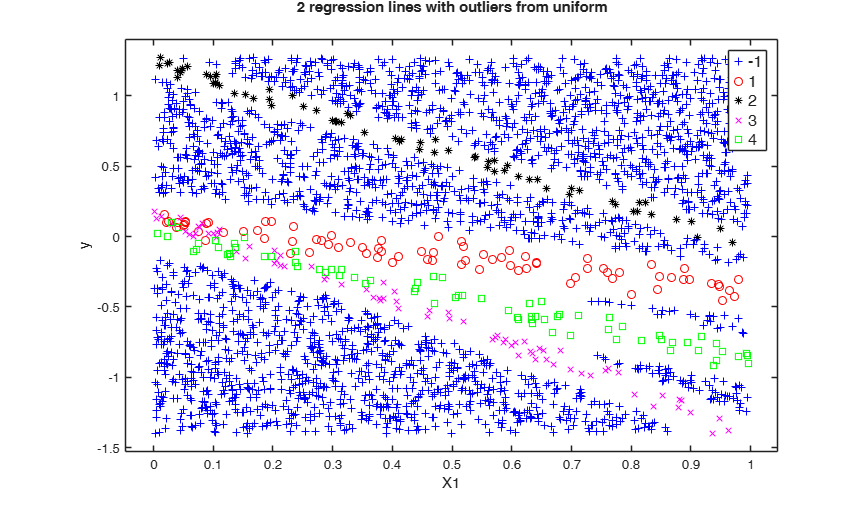###Add outliers generated from Chi2 with 5 degrees of freedom.

n=300;
k=4;
p=2;  % p includes the intercept
out=MixSimreg(k,p,'BarOmega',0.01);
noisevars=0;
noiseunits=struct;
noiseunits.number=3000;
% Add asymmetric very concentrated noise
noiseunits.typeout={'Chisquare5'};
[y,X,id]=simdatasetreg(n, out.Pi, out.Beta, out.S, out.Xdistrib,'noisevars',noisevars,'noiseunits',noiseunits);
[H,AX,BigAx]=yXplot(y,X,'group',id);
title(BigAx,'2 groups with outliers from $\chi^2_5$','Interpreter','Latex')
Warning: it was not possible to generate 3000 outliers
in 30000 replicates in the interval [1--1]
Number of values which was possible to generate is equal to 2848
Please modify the type of outliers using option 'typeout'
or increase input option 'alpha'
The value of alpha now is 0.001
Outliers have been generated according to Chisquare5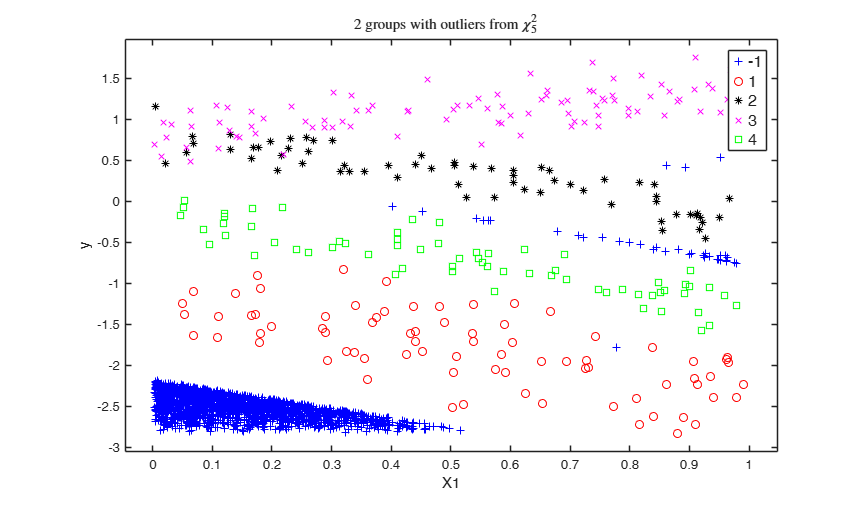## Related Examples

expand all

###Add outliers generated from Chi2 with 40 degrees of freedom.

n=300;
k=4;
p=2;  % p includes the intercept
out=MixSimreg(k,p,'BarOmega',0.01);
noisevars=0;
noiseunits=struct;
noiseunits.number=3000;
% Add asymmetric concentrated noise
noiseunits.typeout={'Chisquare40'};
[y, X,id]=simdatasetreg(n, out.Pi, out.Beta, out.S, out.Xdistrib,'noisevars',noisevars,'noiseunits',noiseunits);
[H,AX,BigAx]=yXplot(y,X,'group',id);
title(BigAx,'4 groups with outliers from $\chi^2_{40}$','Interpreter','Latex')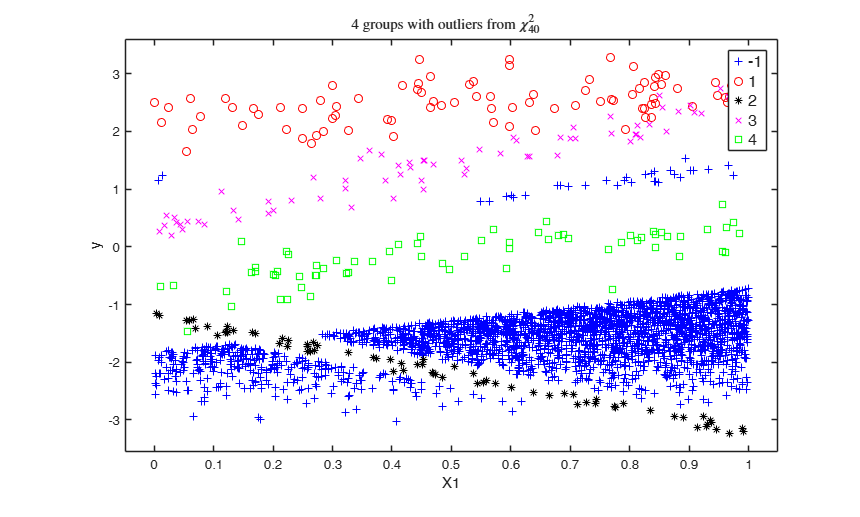###Add outliers generated from normal distribution.

n=300;
k=4;
p=2;  % p includes the intercept
out=MixSimreg(k,p,'BarOmega',0.01);
noisevars=0;
noiseunits=struct;
noiseunits.number=3000;
% Add normal noise
noiseunits.typeout={'normal'};
[y,X,id]=simdatasetreg(n, out.Pi, out.Beta, out.S,out.Xdistrib, 'noisevars',noisevars,'noiseunits',noiseunits);
[H,AX,BigAx]=yXplot(y,X,'group',id);
title(BigAx,'4 groups with outliers from normal distribution','Interpreter','Latex')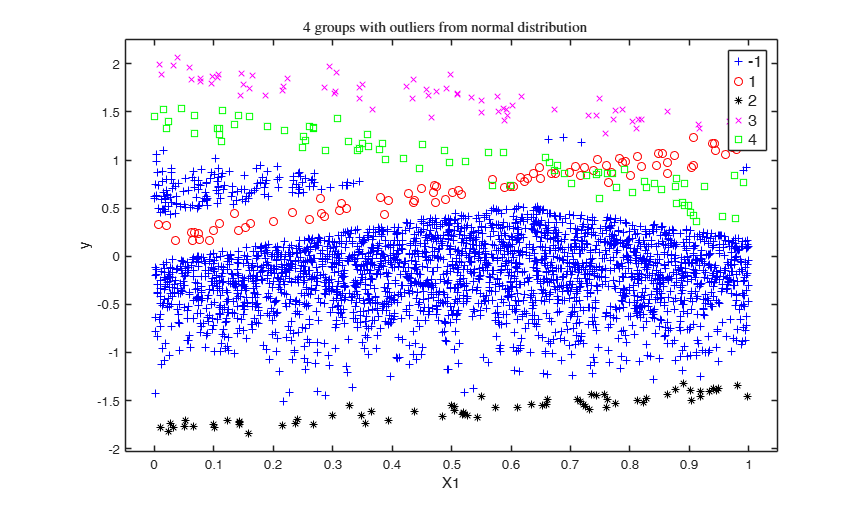###Add outliers generated from Student T with 5 degrees of freedom.

n=300;
k=4;
p=2;  % p includes the intercept
out=MixSimreg(k,p,'BarOmega',0.01);
noisevars=0;
noiseunits=struct;
noiseunits.number=3000;
% Add outliers from T5
noiseunits.typeout={'T5'};
[y, X,id]=simdatasetreg(n, out.Pi, out.Beta, out.S,out.Xdistrib, 'noisevars',noisevars,'noiseunits',noiseunits);
[H,AX,BigAx]=yXplot(y,X,'group',id);
suplabel('4 groups with outliers from Student T with 5 degrees if freedom','t')
ans =

Axes (suplabel) with properties:

XLim: [0 1]
YLim: [0 1]
XScale: 'linear'
YScale: 'linear'
GridLineStyle: '-'
Position: [0.1055 0.0863 0.8395 0.8787]
Units: 'normalized'

Use GET to show all properties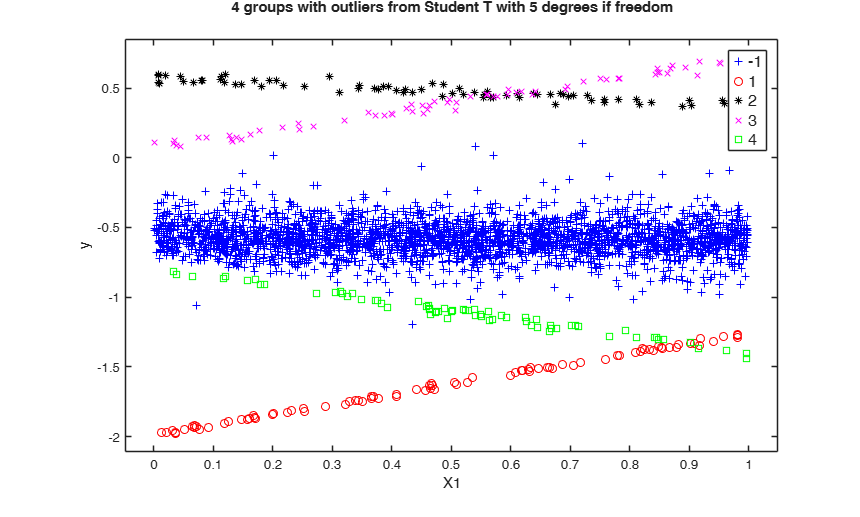###Add componentwise contamination.

n=300;
k=4;
p=2;  % p includes the intercept
out=MixSimreg(k,p,'BarOmega',0.01);
noisevars='';
noiseunits=struct;
noiseunits.number=3000;
% Add asymmetric concentrated noise
noiseunits.typeout={'componentwise'};
[y, X,id]=simdatasetreg(n, out.Pi, out.Beta, out.S,out.Xdistrib, 'noisevars',noisevars,'noiseunits',noiseunits);
yXplot(y,X,'group',id);
suplabel('4 groups with component wise outliers','t')
ans =

Axes (suplabel) with properties:

XLim: [0 1]
YLim: [0 1]
XScale: 'linear'
YScale: 'linear'
GridLineStyle: '-'
Position: [0.1055 0.0863 0.8395 0.8787]
Units: 'normalized'

Use GET to show all properties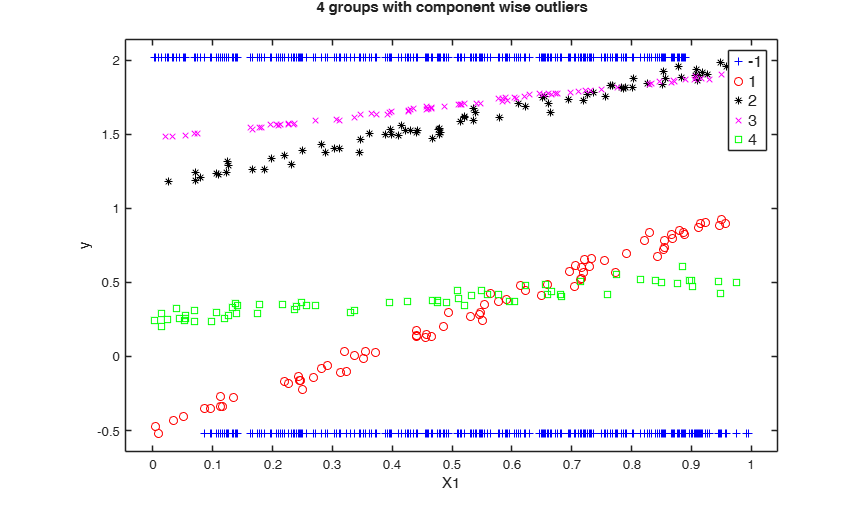###Add outliers generated from Chisquare and T distribution.

n=300;
k=4;
p=2;  % p includes the intercept
out=MixSimreg(k,p,'BarOmega',0.01);
noisevars=0;
noiseunits=struct;
noiseunits.number=5000*ones(2,1);
noiseunits.typeout={'Chisquare3','T20'};
[y, X,id]=simdatasetreg(n, out.Pi, out.Beta, out.S, out.Xdistrib, 'noisevars',noisevars,'noiseunits',noiseunits);
[H,AX,BigAx]=yXplot(y,X,'group',id);
title(BigAx,'4 groups with outliers from $\chi^2_{3}$ and $T_{20}$','Interpreter','Latex')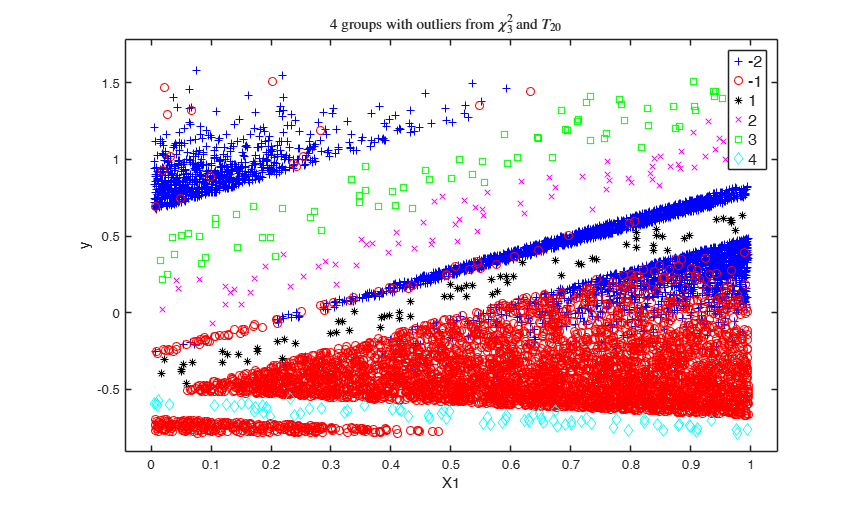###Add outliers from Chisquare and T distribution and use a personalized value of alpha.

n=300;
k=4;
p=2;  % p includes the intercept
out=MixSimreg(k,p,'BarOmega',0.01);
noisevars=0;
noiseunits=struct;
noiseunits.number=5000*ones(2,1);
noiseunits.typeout={'Chisquare3','T20'};
noiseunits.alpha=0.002;
[y, X,id]=simdatasetreg(n, out.Pi, out.Beta, out.S, out.Xdistrib, 'noisevars',noisevars,'noiseunits',noiseunits);
[H,AX,BigAx]=yXplot(y,X,'group',id);
title(BigAx,'4 groups with outliers from $\chi^2_{3}$ and $T_{20}$','Interpreter','Latex')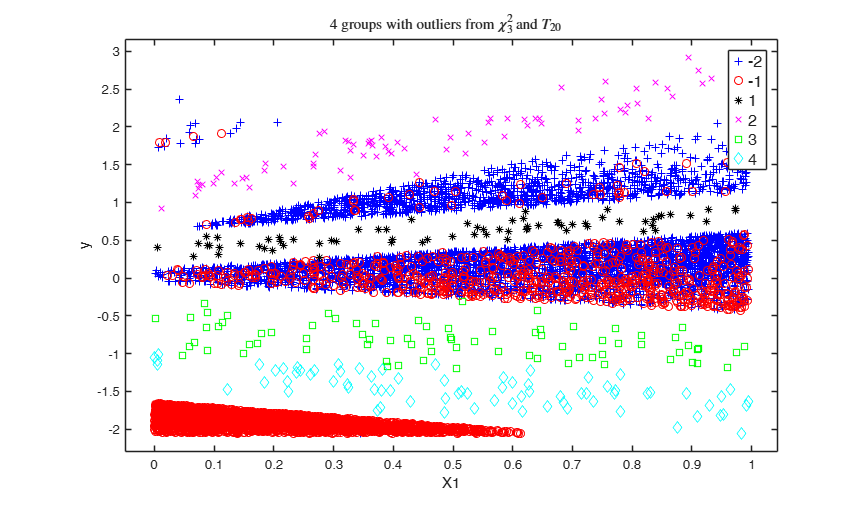###Add outliers from Chi2 and point mass contamination and add one noise variable.

n=300;
k=4;
p=2;  % p includes the intercept
out=MixSimreg(k,p,'BarOmega',0.01);
noisevars=struct;
noisevars.number=1;
noiseunits=struct;
noiseunits.number=[100 100];
noiseunits.typeout={'pointmass' 'Chisquare5'};
[y, X,id]=simdatasetreg(n, out.Pi, out.Beta, out.S, out.Xdistrib, 'noisevars',noisevars,'noiseunits',noiseunits);
[H,AX,BigAx]=yXplot(y,X,'group',id);
title(BigAx,'4 groups with outliers from $\chi^2_{5}$ and point mass $+1$ noise var','Interpreter','Latex')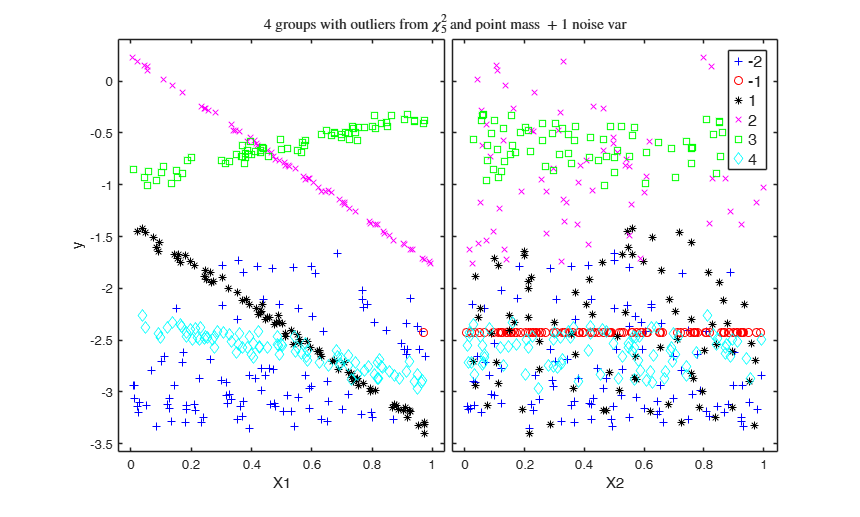###Example of the use of personalized interval to generate outliers.

n=300;
k=4;
p=2;  % p includes the intercept
out=MixSimreg(k,p,'BarOmega',0.01);
noiseunits=struct;
noiseunits.number=1000;
noiseunits.typeout={'uniform'};
% Generate outliers in the interval [-1 1] for the first variable and
% interval [1 2] for the second variable
noiseunits.interval=[-1 1;
1 2];
% Finally add a noise variable
noisevars=struct;
noisevars.number=1;
[y, X,id]=simdatasetreg(n, out.Pi, out.Beta, out.S, out.Xdistrib, 'noisevars',noisevars,'noiseunits',noiseunits);
[H,AX,BigAx]=yXplot(y,X,'group',id);
title(BigAx,'4 groups with outliers from uniform using a personalized interval $+1$ noise var','Interpreter','Latex')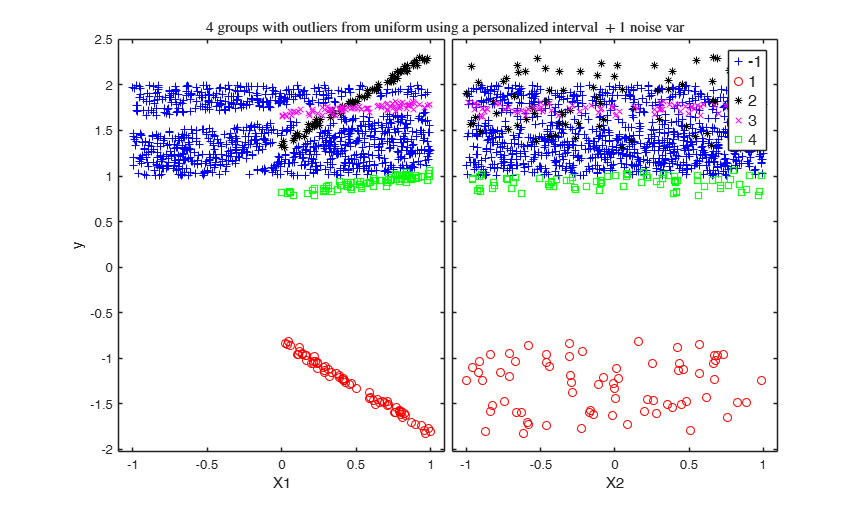### Example of the use of personalized interval to generate outliers (1).

Generate 1000 outliers from uniform in the interval [-2 3] and 1000 units using componentwise contamination in the interval [-2 3]

n=300;
k=4;
p=2;  % p includes the intercept
out=MixSimreg(k,p,'BarOmega',0.01);
noiseunits=struct;
noiseunits.number=[1000 1000];
noiseunits.typeout={'uniform' 'componentwise'};
noiseunits.interval=[-2; 3];
% Finally add a noise variable
noisevars=struct;
noisevars.number=1;
[y, X,id]=simdatasetreg(n, out.Pi, out.Beta, out.S, out.Xdistrib, 'noisevars',noisevars,'noiseunits',noiseunits);
yXplot(y,X,'group',id);
suplabel('4 groups with outliers componentwise and from uniform in the interval [-2 3]','t')

###Example with user defined explanatory variables values (1).

Here the X distribution is the same for each component.

clear all
close all
rng(1234,'twister');
% mixture parameters
intercept = 0; % 1/0 = intercept yes/no
p=1+intercept;
k=2;
n=200;
% beta distributed as halfnormal
% explanatory variables distribution chosen by the User from a beta
XdistribB=struct;
XdistribB.intercept=intercept;
XdistribB.type='User';
X1=random('beta',1,5,n,1);             % data generation: user distribution is a beta
XdistribB.BarX = ones(1,k)*mean(X1);   % mean of the generated data: one per group
% overlap level baromega: chosen at random here, in a given range
mino = 0.01; maxo = 0.1;
baromega = mino + (maxo-mino).*rand(1,1);
% estimated mixsim parameters
% Simulate the data from the mixim parameters and the user values for X
if intercept
Q.Xdistrib.X = [ones(n,1) X1];
else
Q.Xdistrib.X = X1;
end
[y,X,id]=simdatasetreg(n,Q.Pi,Q.Beta,Q.S,Q.Xdistrib);
yXplot(y,X,'group',id,'tag','X_beta');
set(gcf,'Name','X Beta distributed');
title('User-defined distribution for X');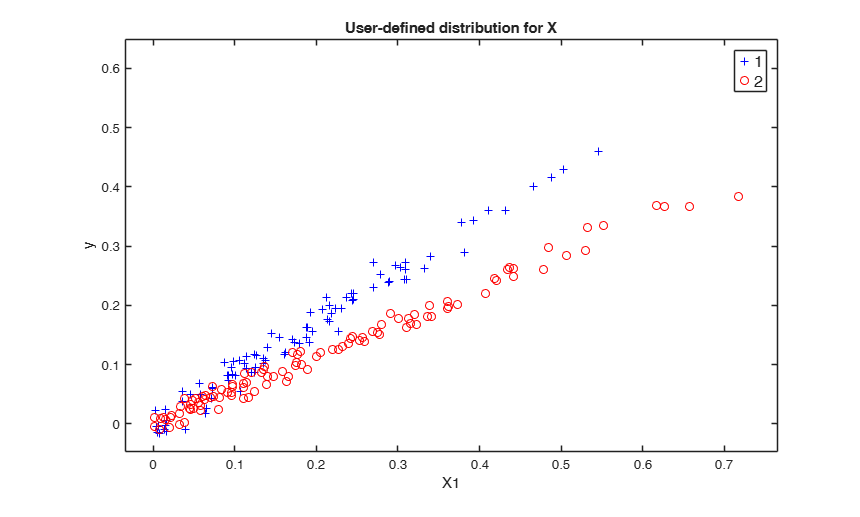###Example with user defined explanatory variables values (2).

Here the X distribution is specific for each component.

clear all
close all
rng(12345,'twister');
% mixture parameters
intercept = 0;      % 1/0 = intercept yes/no
n=200;
p=1+intercept;
k=2;                %do not change k: it would not work (see below to generalise)
% beta distributed as halfnormal
% explanatory variables distribution chosen by the User from a beta
XdistribB=struct;
XdistribB.intercept=intercept;
XdistribB.type='User';
%for i=1:10
% X beta distributed
X2=random('beta',0.5,1,n,1);
muBeta2 = mean(X2);
X1=random('beta',1,0.5,n,1);
muBeta1 = mean(X1);
% data generation: user distribution is a beta
XdistribB.BarX = [muBeta1 muBeta2]; % mean of the generated data: one per group
% overlap level baromega: chosen at random here, in a given range
mino = 0.01; maxo = 0.05;
maxomega = mino + (maxo-mino).*rand(1,1);
% estimated mixsim parameters
% Simulate the data from the mixim parameters and the user values for X
if intercept
Q.Xdistrib.X = [ones(k*n,1) , [X1 ; X2]];
else
Q.Xdistrib.X = [X1 ; X2];
end
[y,X,id]=simdatasetreg(k*n,Q.Pi,Q.Beta,Q.S,Q.Xdistrib);
yXplot(y,X,'group',id,'tag','X_beta');
set(gcf,'Name','X Beta distributed');
title('User-defined distribution for X');## Input Arguments

### n — sample size of the dataset. Scalar.

Data Types: single| double

### Pi — vector of length k defining mixing proportions. Vector.

$\sum_{j=1}^k \pi=1$.

Data Types: single| double

### Beta — p-by-k matrix containing (in the columns) regression coefficients for the k groups. Matrix.

Data Types: single| double

### S — vector of length k containing the variances of the k regression hyperplanes. Vector.

Data Types: single| double

### Xdistrib — information about how to generate each explanatory variable inside each group. Structure.

Structure which contains the following fields:

Value Description
intercept

scalar equal to 1 if intercept is present. The default value of Xdistrib.intercept is 1.

type

type of distribution. Possible values for are 'normal', 'halfnormal', 'uniform', 'User'.

mu

matrix of size (p-1)-by-k if (Xdistrib.intercept=1) or p-by-k if (Xdistrib.intercept=0) containing the parameters mu for each explanatory variable and each group. The default value of Xdistrib.mu is zeros(p-1, k). Note that Xdistrib.mu is used just if Xdistrib.type is

sigma

matrix of size (p-1)-by-k if (Xdistrib.intercept=1) or p-by-k if (Xdistrib.intercept=0) containing the parameters sigma for each explanatory variable and each group.

The default value of Xdistrib.sigma is ones(p-1,k).

Notethat Xdistrib.sigma is used just if Xdistrib.type is 'normal' or 'halfnormal'.

a

matrix of size (p-1)-by-k if (Xdistrib.intercept=1) or p-by-k if (Xdistrib.intercept=0) containing the parameters a for each explanatory variable and each group. The default value of Xdistrib.a is zeros(p-1, k).

Notethat Xdistrib.a is used just if Xdistrib.type is 'uniform', that is if we have U(a, b).

b

matrix of size (p-1)-by-k if (Xdistrib.intercept=1) or p-by-k if (Xdistrib.intercept=0) containing the parameters b for each explanatory variable and each group. The default value of Xdistrib.a is zeros(p-1, k).

Note that Xdistrib.b is used just if Xdistrib.type is 'uniform'; that is we have U(a, b).

X

matrix with at least n rows and p-1 (if intercept is present) or p (if intercept is not present) columns containing the values of the explanatory variables for the k groups.

Notethat Xdistrib.X is used just if Xdistrib.type is 'User'.

Data Types: struct

### Name-Value Pair Arguments

Specify optional comma-separated pairs of Name,Value arguments. Name is the argument name and Value is the corresponding value. Name must appear inside single quotes (' '). You can specify several name and value pair arguments in any order as  Name1,Value1,...,NameN,ValueN.

Example:  'noiseunits', 10 , 'noisevars', 5 , 'lambda',2; 

### noiseunits —number of type of outlying observations.scalar | structure.

Missing value, scalar or structure.

This input parameter specifies the number and type of outlying observations. The default value of noiseunits is 0.

- If noiseunits is a scalar t different from 0, then t units from the uniform distribution in the interval min([X y]) max([X y]) are generated in such a way that their squared distance from the fitted value (squared residual) of each existing group is larger then the quantile 1-0.999 of the Chi^2 distribution with 1 degree of freedom. In order to generate these units the maximum number of attempts is equal to 10000.

- If noiseunits is a structure it may contain the following fields:

number = scalar, or vector of length f. The sum of the elements of vector 'number' is equal to the total number of outliers which are simulated.

alpha = scalar or vector of legth f containing the level(s) of simulated outliers. The default value of alpha is 0.001.

maxiter = maximum number of trials to simulate outliers.

The default value of maxiter is 10000.

interval= missing value or vector of length 2, or matrix of size 2-by-2 or matrix of size 2-by-(p+1) which controls the min and max of the generated outliers for each dimension.

* If interval is a vector of length 2 each outlier has a value for each column of X and y which lies inside interval(1) and interval(2).

* If interval is a matrix of size 2-by-2 each outlier has a value for each column of X which lies inside interval(1,1) and interval(2,1) and a value of y which lies inside interval(1,2) and interval(2,2).

* If interval is a 2-by-(p+1) matrix outliers are simulated in:

interval(1,1) interval (2,1) for expl variable 1 ...

interval(1,p) interval (2,p) for expl variable p interval(1,p+1) interval (2,p+1) for response y.

If interval is empty (default), the outliers are simulated in the interval min(X) max(X) and min(y) max (y).

typeout = list of length f containing the type of outliers which must be simulated. Possible values for typeout are:

* unif (or uniform), if the outliers must be generated using the uniform distribution;

* norm (or normal), if the outliers must be generated using the normal distribution;

* Chisquarez, if the outliers must be generated using the Chi2 distribution with z degrees of freedom;

* Tz or tz, if the outliers must be generated using the Student T distribution with z degrees of freedom;

* pointmass, if the outliers are concentrated on a particular point;

* by_comp, if the outliers are distributed along a linear component. The option was introduced to add dense area in one linear component.

* componentwise, if the outliers must have the same coordinates of the existing rows of matrix X apart from the single coordinate of y (which will be the min or max of y or to the min or max specified in interval).

For example, the code:

noiseunits=struct;

Value Description
number

[100 100];

typeout

{'uniform' 'componentwise'};

interval

[-2 2];

adds 200 outliers, the first 100 generated using a uniform distribution and the last 100 using componentwise scheme. Outliers are generated in the interval [-2 2] for each variable.

Example:  'noiseunits', 10 

Data Types: double

### noisevars —Type of noise explanatory variables.scalar | structure.

Empty value, scalar or structure.

- If noisevars is not specified or is an empty value (default) no noise variable is added to the matrix of simulated data.

- If noisevars is a scalar equal to r, then r new noise explnatory variables are added to the matrix of simulated data using the uniform distribution in the range [min(X) max(X)].

- If noisevars is a structure it may contain the following fields:

Value Description
number

a scalar or a vector of length f. The sum of elements of vector 'number' is equal to the total number of noise variables to be addded.

distribution

string or cell array of strings of length f which specifies the distribution to be used to simulate the noise variables.

If field distribution is not present then the uniform distribution is used to simulate the noise variables.

String 'distribution' can be one of the following values:

* uniform = uniform distribution * normal = normal distribution * t or T followed by a number which controls the degrees of freedom. For example, t6 specifies to generate the data according to a Student T with 6 degrees of freedom.

* chisquare followed by a number which controls the degreess of freedom. For example, chisquare8 specifies to generate the data according to a Chi square distribution with 8 degrees of freedom.

interval

string or vector of length 2 or matrix of size 2-by-f (where f is the number of noise variables) which controls for each element of vector 'number' or each element of cell 'distribution', the min and max of the noise variables. For example, interval(1,3) and interval(2,3) are respectively the minimum and maximum values of simulated the data for the third noise variable If interval is empty (default), the noise variables are simulated uniformly between the smallest and the largest coordinates of the simulated data matrix X.

For example, the code:

noisevars=struct;

noisevars.number=[3 2];

noisevars.distribution={'Chisquare5' 'T3'};

adds 5 noise explaantory variables, the first 3 generated using the Chi2 with 5 degrees of freedom and the last two using the Student t with 3 degrees of freedom. Noise variables are generated in the interval min(X) max(X).

Example:  'noisevars', 5 

Data Types: double

### lambda —Transformation coefficient.scalar.

Scalar containing inverse Box-Cox transformation coefficient to apply to y.

The value false (default) implies that no transformation is applied to response variable.

Example:  'lambda',2; 

Data Types: double

## Output Arguments

### y —Response variable.  Vector

Vector of dimension (n+nout)-by-1 containing the values of the responses for the k groups.

### X —Explanatory variables.  Matrix

Matrix of size (n + nout)-by-(p + nnoise) containinng the values of the explanatory variables for the k groups. Noise coordinates are provided in the last nnoise columns.

### id —classification vector.  Vector

Classification vector of length n + nout; 0 represents an outlier.

REMARK: If nout outliers could not be generated a warning is produced. In this case matrix X and vector id will have just n rows.